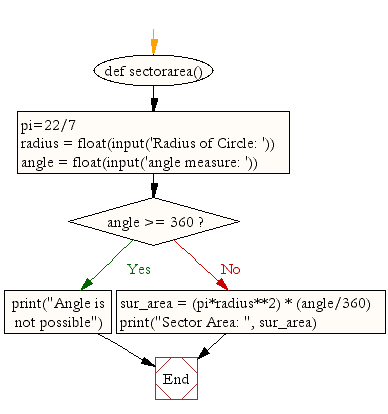﻿ Python Math: Calculate the area of a sector - w3resource# Python Math: Calculate the area of sector

## Python Math: Exercise-8 with Solution

Write a Python program to calculate the area of a sector.
Note: A circular sector or circle sector, is the portion of a disk enclosed by two radii and an arc, where the smaller area is known as the minor sector and the larger being the major sector.

Sample Solution:-

Python Code:

``````def sectorarea():
pi=22/7
angle = float(input('angle measure: '))
if angle >= 360:
print("Angle is not possible")
return
print("Sector Area: ", sur_area)

sectorarea()
```
```

Sample Output:

```Radius of Circle: 4
angle measure: 45
Sector Area:  6.285714285714286
```

Pictorial Presentation:Flowchart:## Visualize Python code execution:

The following tool visualize what the computer is doing step-by-step as it executes the said program:

Python Code Editor:

Have another way to solve this solution? Contribute your code (and comments) through Disqus.

What is the difficulty level of this exercise?

Test your Programming skills with w3resource's quiz.

﻿

## Python: Tips of the Day

Performs right-to-left function composition:

Example:

```from functools import reduce

def tips_compose(*fns):
return reduce(lambda f, g: lambda *args: f(g(*args)), fns)
add6 = lambda x: x + 6
multiply = lambda x, y: x * y
```36